Download the eSaral app and start learning from Kota's top IITians and doctors.

# NCERT Solutions for Class 11 Chemistry chapter 9 Hydrogen PDF - eSaral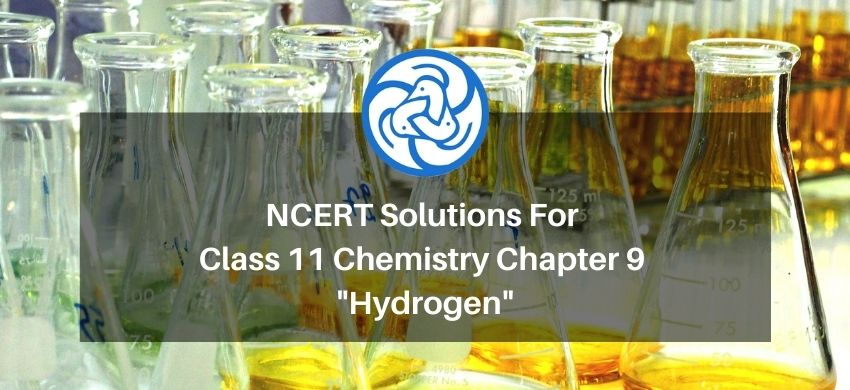# NCERT Solutions for Class 11 Chemistry chapter 9 Hydrogen PDF

Hey, are you a class 11 student and looking for ways to download NCERT Solutions for Class 11 Chemistry chapter 9 Hydrogen PDF? If yes. Then read this post till the end.

In this article, we have listed NCERT Solutions for Class 11 Chemistry chapter 9 Hydrogen in PDF that are prepared by Kota’s top IITian’s Faculties by keeping Simplicity in mind.

If you want to learn and understand class 11 Chemistry chapter 9 "Hydrogen" in an easy way then you can use these solutions PDF.

NCERT Solutions helps students to Practice important concepts of subjects easily. Class 11 Chemistry solutions provide detailed explanations of all the NCERT questions that students can use to clear their doubts instantly.

If you want to score high in your class 11 Chemistry Exam then it is very important for you to have a good knowledge of all the important topics, so to learn and practice those topics you can use eSaral NCERT Solutions.

So, without wasting more time Let’s start.

### Download NCERT Solutions for Class 11 Chemistry chapter 9 Hydrogen PDF

Question 1:Justify the position of hydrogen in the periodic table on the basis of its electronic configuration.

Solution. Hydrogen is the first element of the periodic table. Its electronic configuration is $\left[1 s^{1}\right]$. Due to the presence of only one electron in its $1 \mathrm{~s}$ shell,

hydrogen exhibits a dual behaviour, i.e., it resembles both alkali metals and halogens.

Resemblance with alkali metals:

1. Like alkali metals, hydrogen contains one valence electron in its valency shell.

$\mathrm{H}: 1 \mathrm{~s}^{1}$

$\mathrm{Li}:[\mathrm{He}] 2 s^{1}$

$\mathrm{Na}:[\mathrm{Ne}] 3 s^{1}$

Hence, it can lose one electron to form a unipositive ion.

2. Like alkali metals, hydrogen combines with electronegative elements to form oxides, halides, and sulphides.

Resemblance with halogens:

1. Both hydrogen and halogens require one electron to complete their octets.

$\mathrm{H}: 1 s^{1}$

$\mathrm{F}: 1 \mathrm{~s}^{2} 2 \mathrm{~s}^{2} 2 p^{5}$

$\mathrm{Cl}: 1 s^{2} 2 s^{2} 2 p^{6} 3 s^{2} 3 p^{5}$

Hence, hydrogen can gain one electron to form a uninegative ion.

2. Like halogens, it forms a diatomic molecule and several covalent compounds.

Though hydrogen shows some similarity with both alkali metals and halogens, it differs from them on some grounds. Unlike alkali metals. hydrogen does not possess metallic characteristics. On the other hand, it possesses a high ionization enthalpy. Also, it is less reactive than halogens.

Owing to these reasons, hydrogen cannot be placed with alkali metals (group I) or with halogens (group VII). In addition, it was also established that $\mathrm{H}^{+}$ ions cannot exist freely as they are extremely small. $\mathrm{H}^{+}$ ions are always associated with other atoms or molecules. Hence, hydrogen is best placed separately in the periodic table.

Question 2:Write the names of isotopes of hydrogen. What is the mass ratio of these isotopes?

Solution. Hydrogen has three isotopes. They are:

2. Deuterium, ${ }_{1}^{2} \mathrm{H}$ or $\mathrm{D}$, and

3. Tritium, ${ }_{1}^{3} \mathrm{H}$ or $\mathrm{T}$

The mass ratio of protium, deuterium and tritium is $1: 2: 3$.

Question 3:Why does hydrogen occur in a diatomic form rather than in a monoatomic form under normal conditions?

Solution. The ionization enthalpy of hydrogen atom is very high $\left(1312 \mathrm{~kJ} \mathrm{~mol}^{-1}\right)$. Hence, it is very hard to remove its only electron. As a result, its tendency to exist in the monoatomic form is rather low. Instead, hydrogen forms a covalent bond with another hydrogen atom and exists as a diatomic $\left(\mathrm{H}_{2}\right)$ molecule.

Question 4:How can the production of dihydrogen, obtained from 'coal gasification', be increased?

Solution. Dihydrogen is produced by coal gasification method as:

$\mathrm{C}_{(s)}+\mathrm{H}_{2} \mathrm{O}_{(g)} \stackrel{1270 \mathrm{~K}}{\longrightarrow} \mathrm{CO}_{(g)}+\mathrm{H}_{2(g)}$

(coal)

The yield of dihydrogen (obtained from coal gasification) can be increased by reacting carbon monoxide (formed during the reaction) with steam in the presence of iron chromate as a catalyst.

$\mathrm{CO}_{(g)}+\mathrm{H}_{2} \mathrm{O}_{(g)} \underset{\text { Caallst }}{673 \mathrm{~K}}{\longrightarrow} \mathrm{CO}_{2(g)}+\mathrm{H}_{2(g)}$

This reaction is called the water-gas shift reaction. Carbon dioxide is removed by scrubbing it with a solution of sodium arsenite.

Question 5:Describe the bulk preparation of dihydrogen by electrolytic method. What is the role of an electrolyte in this process?

Solution. Dihydrogen is prepared by the electrolysis of acidified or alkaline water using platinum electrodes. Generally, $15-20 \%$ of an acid $\left(\mathrm{H}_{2} \mathrm{SO}_{4}\right)$ or a base $(\mathrm{NaOH})$ is used.

Reduction of water occurs at the cathode as:

$2 \mathrm{H}_{2} \mathrm{O}+2 \mathrm{e}^{-} \longrightarrow 2 \mathrm{H}_{2}+2 \mathrm{OH}^{-}$

At the anode, oxidation of $\mathrm{OH}^{-}$ ions takes place as:

$2 \mathrm{OH}^{-} \longrightarrow \mathrm{H}_{2} \mathrm{O}+\frac{1}{2} \mathrm{O}_{2}+2 \mathrm{e}^{-}$

$\therefore$ Net reaction can be represented as:

$\mathrm{H}_{2} \mathrm{O}_{(i)} \longrightarrow \mathrm{H}_{2(g)}+\frac{1}{2} \mathrm{O}_{2(g)}$

Electrical conductivity of pure water is very low owing to the absence of ions in it. Therefore, electrolysis of pure water also takes place at a low rate. If an electrolyte such as an acid or a base is added to the process, the rate of electrolysis increases. The addition of the electrolyte

makes the ions available in the process for the conduction of electricity and for electrolysis to take place.

Question 6:Complete the following reactions:

(i) $\mathrm{H}_{2(g)}+\mathrm{M}_{\mathrm{m}} \mathrm{O}_{\mathrm{o}(s)} \stackrel{\Delta}{\longrightarrow}$

(ii) $\mathrm{CO}_{(\mathrm{g})}+\mathrm{H}_{2(\mathrm{~g})} \frac{\Delta}{\text { catalyst }}$

(iii) $\mathrm{C}_{3} \mathrm{H}_{8(g)}+3 \mathrm{H}_{2} \mathrm{O}_{(g)} \underset{\text { caalyst }}{ }$

(iv) $\mathrm{Zn}_{(s)}+\mathrm{NaOH}_{(a q)} \stackrel{\text { heat }}{\longrightarrow}$

Solution. (i) $\mathrm{H}_{2(g)}+\mathrm{M}_{\mathrm{m}} \mathrm{O}_{o(s)} \stackrel{\Delta}{\longrightarrow} \mathrm{mM}_{(s)}+\mathrm{H}_{2} \mathrm{O}_{(f)}$

(ii) $\mathrm{CO}_{(g)}+2 \mathrm{H}_{2(g)} \underset{\text { cualyst }}{\longrightarrow} \mathrm{CH}_{3} \mathrm{OH}_{(l)}$

(iii) $\mathrm{C}_{3} \mathrm{H}_{8(g)}+3 \mathrm{H}_{2} \mathrm{O}_{(g)} \underset{\text { catalyst }}{\longrightarrow} 3 \mathrm{CO}_{(g)}+7 \mathrm{H}_{2(g)}$

(iv) $\mathrm{Zn}_{(s)}+2 \mathrm{NaOH}_{(q q)}$

$\stackrel{\text { heat }}{\longrightarrow} \mathrm{Na}_{2} \mathrm{ZnO}_{2(\text { aq) }}+\mathrm{H}_{2(g)}$

Sodium zincate

Question 7.Discuss the consequences of high enthalpy of H-H bond in terms of chemical reactivity of dihydrogen.

Solution. The ionization enthalpy of $\mathrm{H}-\mathrm{H}$ bond is very high $\left(1312 \mathrm{~kJ} \mathrm{~mol}^{-1}\right)$. This indicates that hydrogen has a low tendency to form $\mathrm{H}^{+}$ ions. Its ionization enthalpy value is comparable to that of halogens. Hence, it forms diatomic molecules $\left(\mathrm{H}_{2}\right)$, hydrides with elements, and a large number of covalent bonds.

Since ionization enthalpy is very high, hydrogen does not possess metallic characteristics (lustre, ductility, etc.) like metals.

Question 8:What do you understand by (i) electron-deficient, (ii) electron-precise, and (iii) electron-rich compounds of hydrogen? Provide justification with suitable examples.

Solution. Molecular hydrides are classified on the basis of the presence of the total number of electrons and bonds in their Lewis structures as:

1. Electron-deficient hydrides

2. Electron-precise hydrides

3. Electron-rich hydrides

An electron-deficient hydride has very few electrons, less than that required for representing its conventional Lewis structure e.g. diborane $\left(\mathrm{B}_{2} \mathrm{H}_{6}\right) .$ In $\mathrm{B}_{2} \mathrm{H}_{6}$, there are six bonds in all, out of which only four bonds are regular two centered-two electron bonds. The remaining two bonds are three centered-two electron bonds i.e., two electrons are shared by three atoms. Hence, its conventional Lewis structure cannot be drawn.

An electron-precise hydride has a sufficient number of electrons to be represented by its conventional Lewis structure e.g. $\mathrm{CH}_{4}$. The Lewis structure can be written as: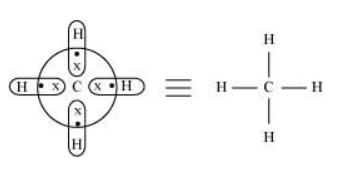Four regular bonds are formed where two electrons are shared by two atoms.

An electron-rich hydride contains excess electrons as lone pairs e.g. $\mathrm{NH}_{3}$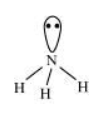There are three regular bonds in all with a lone pair of electrons on the nitrogen atom.

Question 9: What characteristics do you expect from an electron-deficient hydride with respect to its structure and chemical reactions?

Solution. An electron-deficient hydride does not have sufficient electrons to form a regular bond in which two electrons are shared by two atoms e.g. $\mathrm{B}_{2} \mathrm{H}_{6}, \mathrm{Al}_{2} \mathrm{H}_{6}$ etc.

These hydrides cannot be represented by conventional Lewis structures. $\mathrm{B}_{2} \mathrm{H}_{6}$, for example, contains four regular bonds and two three centered-two electron bond. Its structure can be represented as: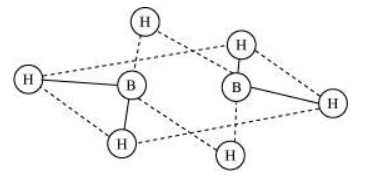Since these hydrides are electron-deficient, they have a tendency to accept electrons. Hence, they act as Lewis acids.

$\mathrm{B}_{2} \mathrm{H}_{6}+2 \mathrm{NMe} \longrightarrow 2 \mathrm{BH}_{3} \cdot \mathrm{NMe}_{3}$

$\mathrm{B}_{2} \mathrm{H}_{6}+2 \mathrm{CO} \longrightarrow 2 \mathrm{BH}_{3} \cdot \mathrm{CO}$

Question 10: Do you expect the carbon hydrides of the type $\left(\mathrm{C}_{n} \mathrm{H}_{2 n+2}\right)$ to act as 'Lewis' acid or base? Justify your answer.

Solution. For carbon hydrides of type $\mathrm{C}_{n} \mathrm{H}_{2 n+2}$, the following hydrides are possible for

$n=1 \Rightarrow \mathrm{CH}_{4}$

$n=2 \Rightarrow \mathrm{C}_{2} \mathrm{H}_{6}$

$n=3 \Rightarrow \mathrm{C}_{3} \mathrm{H}_{8}$

$\vdots \quad \vdots$

For a hydride to act as a Lewis acid i.e., electron accepting, it should be electron-deficient. Also, for it to act as a Lewis base i.e., electron donating, it should be electron-rich.

Taking $\mathrm{C}_{2} \mathrm{H}_{6}$ as an example, the total number of electrons are 14 and the total covalent bonds are seven. Hence, the bonds are regular $2 \mathrm{e}^{-}-2$ centered bonds.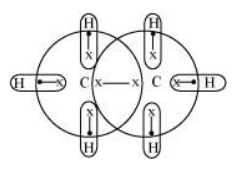Hence, hydride $\mathrm{C}_{2} \mathrm{H}_{6}$ has sufficient electrons to be represented by a conventional Lewis structure. Therefore, it is an electron-precise hydride, having all atoms with complete octets. Thus, it can neither donate nor accept electrons to act as a Lewis acid or Lewis base.

Question 11:What do you understand by the term "non-stoichiometric hydrides"? Do you expect this type of the hydrides to be formed by alkali metals? Justify your answer.

Solution. Non-Stoichiometric hydrides are hydrogen-deficient compounds formed by the reaction of dihydrogen with $d$ -block and $f$ -block elements. These hydrides do not follow the law of constant composition. For example: $\mathrm{LaH}_{2.87}, \mathrm{YbH}_{2.55}, \mathrm{TiH}_{1.5-1.8}$ etc.

Alkali metals form stoichiometric hydrides. These hydrides are ionic in nature. Hydride ions have comparable sizes ( $208 \mathrm{pm}$ ) with alkali metal ions. Hence, strong binding forces exist between the constituting metal and hydride ion. As a result, stoichiometric hydrides are formed.

Alkali metals will not form non-stoichiometric hydrides.

Question 12:How do you expect the metallic hydrides to be useful for hydrogen storage? Explain.

Solution. Metallic hydrides are hydrogen deficient, i.e., they do not hold the law of constant composition. It has been established that in the hydrides of Ni, Pd, Ce, and Ac, hydrogen occupies the interstitial position in lattices allowing further absorption of hydrogen on these metals. Metals like Pd, Pt, etc. have the capacity to accommodate a large volume of hydrogen. Therefore, they are used for the storage of hydrogen and serve as a source of energy.

Question 13:How does the atomic hydrogen or oxy-hydrogen torch function for cutting and welding purposes? Explain.

Solution. Atomic hydrogen atoms are produced by the dissociation of dihydrogen with the help of an electric arc. This releases a huge amount of energy $\left(435.88 \mathrm{~kJ} \mathrm{~mol}^{-1}\right)$. This energy can be used to generate a temperature of $4000 \mathrm{~K}$, which is ideal for welding and cutting metals. Hence, atomic hydrogen or oxy-hydrogen torches are used for these purposes. For this reason, atomic hydrogen is allowed to recombine on the surface to be welded to generate the desired temperature.

Question 14:Among $\mathrm{NH}_{3}, \mathrm{H}_{2} \mathrm{O}$ and $\mathrm{HF}$, which would you expect to have highest magnitude of hydrogen bonding and why?

Solution. The extent of hydrogen bonding depends upon electronegativity and the number of hydrogen atoms available for bonding. Among nitrogen, fluorine, and oxygen, the increasing order of their electronegativities are $\mathrm{N}<\mathrm{O}<\mathrm{F}$.

Hence, the expected order of the extent of hydrogen bonding is $\mathrm{HF}>\mathrm{H}_{2} \mathrm{O}>\mathrm{NH}_{3}$.

But, the actual order is $\mathrm{H}_{2} \mathrm{O}>\mathrm{HF}>\mathrm{NH}_{3}$.

Although fluorine is more electronegative than oxygen, the extent of hydrogen bonding is higher in water. There is a shortage of hydrogens in HF, whereas there are exactly the right numbers of hydrogens in water. As a result, only straight chain bonding takes place. On the other hand, oxygen forms a huge ring-like structure through its high ability of hydrogen bonding.

In case of ammonia, the extent of hydrogen bonding is limited because nitrogen has only one lone pair. Therefore, it cannot satisfy all hydrogens.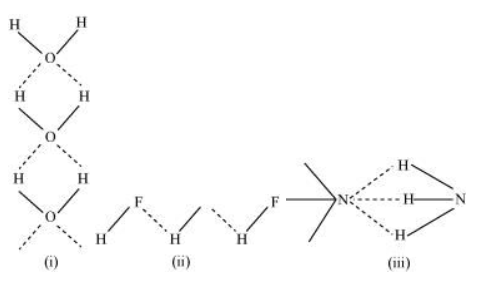Question 15:Saline hydrides are known to react with water violently producing fire. Can $\mathrm{CO}_{2}$, a well known fire extinguisher, be used in this case? Explain.

Solution. Saline hydrides (i.e., NaH, LiH, etc.) react with water to form a base and hydrogen gas. The chemical equation used to represent the reaction can be written as:

$\mathrm{MH}_{(x)}+\mathrm{H}_{2} \mathrm{O}_{(a q)} \longrightarrow \mathrm{MOH}_{(\alpha q)}+\mathrm{H}_{2(g)}$

The reaction is violent and produces fire.

$\mathrm{CO}_{2}$ is heavier than dioxygen. It is used as a fire extinguisher because it covers the fire as a blanket and inhibits the supply of dioxygen, thereby dousing the fire.

$\mathrm{CO}_{2}$ can be used in the present case as well. It is heavier than dihydrogen and will be effective in isolating the burning surface from dihydrogen and dioxygen.

Question 16:Arrange the following

(i) $\mathrm{CaH}_{2}, \mathrm{BeH}_{2}$ and $\mathrm{TiH}_{2}$ in order of increasing electrical conductance.

(ii) LiH, $\mathrm{NaH}$ and CsH in order of increasing ionic character.

(iii) H-H, D-D and F-F in order of increasing bond dissociation enthalpy.

(iv) $\mathrm{NaH}, \mathrm{MgH}_{2}$ and $\mathrm{H}_{2} \mathrm{O}$ in order of increasing reducing property.

Solution. (i) The electrical conductance of a molecule depends upon its ionic or covalent nature. Ionic compounds conduct, whereas covalent compounds do not.

$\mathrm{BeH}_{2}$ is a covalent hydride. Hence, it does not conduct. $\mathrm{CaH}_{2}$ is an ionic hydride, which conducts electricity in the molten state. Titanium hydride, $\mathrm{TiH}_{2}$ is metallic in nature and conducts electricity at room temperature. Hence, the increasing order of electrical conductance is as follows:

$\mathrm{BeH}_{2}<\mathrm{CaH}_{2}<\mathrm{TiH}_{2}$

(ii) The ionic character of a bond is dependent on the electronegativities of the atoms involved. The higher the difference between the electronegativities of atoms, the smaller is the ionic character. Electronegativity decreases down the group from Lithium to Caesium. Hence, the ionic character of their hydrides will increase (as shown below

$\mathrm{LiH}<\mathrm{NaH}<\mathrm{CsH}$

(iii) Bond dissociation energy depends upon the bond strength of a molecule, which in turn depends upon the attractive and repulsive forces present in a molecule.

The bond pair in D-D bond is more strongly attracted by the nucleus than the bond pair in $\mathrm{H}-\mathrm{H}$ bond. This is because of the higher nuclear mass of $D_{2}$. The stronger the attraction, the greater will be the bond strength and the higher is the bond dissociation enthalpy. Hence, the bond dissociation enthalpy of $D-D$ is higher than $H-H$.

However, bond dissociation enthalpy is the minimum in the case of F-F. The bond pair experiences strong repulsion from the lone pairs present on each F-centre.

Therefore, the increasing order of bond dissociation enthalpy is as follows:

$\mathrm{F}-\mathrm{F}<\mathrm{H}-\mathrm{H}<\mathrm{D}-\mathrm{D}$

(iv) lonic hydrides are strong reducing agents. $\mathrm{NaH}$ can easily donate its electrons. Hence, it is most reducing in nature.

Both, $\mathrm{MgH}_{2}$ and $\mathrm{H}_{2} \mathrm{O}$ are covalent hydrides. $\mathrm{H}_{2} \mathrm{O}$ is less reducing than $\mathrm{MgH}_{2}$ since the bond dissociation energy of $\mathrm{H}_{2} \mathrm{O}$ is higher than $\mathrm{MgH}_{2}$

Hence, the increasing order of the reducing property is $\mathrm{H}_{2} \mathrm{O}<\mathrm{MgH}_{2}<\mathrm{NaH}$.

Question 17:Compare the structures of $\mathrm{H}_{2} \mathrm{O}$ and $\mathrm{H}_{2} \mathrm{O}_{2}$.

Solution. In gaseous phase, water molecule has a bent form with a bond angle of $104.5^{\circ} .$ The $\mathrm{O}-\mathrm{H}$ bond length is $95.7 \mathrm{pm} .$ The structure can be shown as: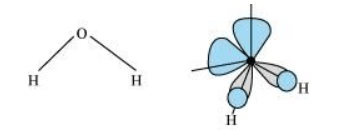Hydrogen peroxide has a non-planar structure both in gas and solid phase. The dihedral angle in gas and solid phase is $111.5^{\circ}$ and $90.2^{\circ}$ respectively.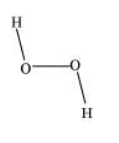Question 18:What do you understand by the term 'auto-protolysis' of water? What is its significance?

Solution. Auto-protolysis (self-ionization) of water is a chemical reaction in which two water molecules react to produce a hydroxide ion $\left(\mathrm{OH}^{-}\right)$ and a hydronium ion $\left(\mathrm{H}_{3} \mathrm{O}^{+}\right)$

The reaction involved can be represented as: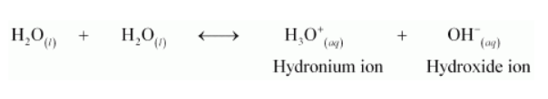Auto-protolysis of water indicates its amphoteric nature i.e., its ability to act as an acid as well as a base.

The acid-base reaction can be written as: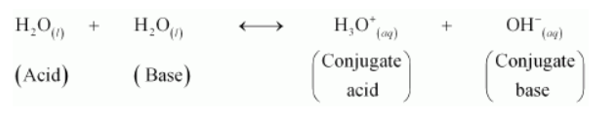Question 19 :Consider the reaction of water with $\mathrm{F}_{2}$ and suggest, in terms of oxidation and reduction, which species are oxidized/reduced.

Solution. The reaction between fluorine and water can be represented as:

$2 \mathrm{~F}_{2(g)}+2 \mathrm{H}_{2} \mathrm{O}_{(t)} \longrightarrow 4 \mathrm{H}_{(a q)}^{+}+4 \mathrm{~F}_{(\text {aq })}^{-}+\mathrm{O}_{2(g)}$

This is an example of a redox reaction as water is getting oxidized to oxygen, while fluorine is being reduced to fluoride ion.

The oxidation numbers of various species can be represented as: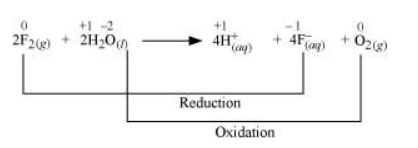Fluorine is reduced from zero to $(-1)$ oxidation state. A decrease in oxidation state indicates the reduction of fluorine.

Water is oxidized from $(-2)$ to zero oxidation state. An increase in oxidation state indicates oxidation of water.

Question 20:Complete the following chemical reactions.

(i) $\mathrm{PbS}_{(s)}+\mathrm{H}_{2} \mathrm{O}_{2(a q)} \rightarrow$

(ii) $\mathrm{MnO}_{4(a q)}^{-}+\mathrm{H}_{2} \mathrm{O}_{(g)} \rightarrow$

(iii) $\mathrm{CaO}_{(s)}+\mathrm{H}_{2} \mathrm{O}_{(g)} \rightarrow$

(iv) $\mathrm{AlCl}_{3(g)}+\mathrm{H}_{2} \mathrm{O}_{(t)} \rightarrow$

(v) $\mathrm{Ca}_{3} \mathrm{~N}_{2(s)}+\mathrm{H}_{2} \mathrm{O}_{(t)} \rightarrow$

Classify the above into (a) hydrolysis, (b) redox and (c) hydration reactions.

Solution. (i) $\mathrm{PbS}_{(s)}+\mathrm{H}_{2} \mathrm{O}_{2(a q)} \rightarrow \mathrm{PbSO}_{4(s)}+\mathrm{H}_{2} \mathrm{O}_{(i)}$

$\mathrm{H}_{2} \mathrm{O}_{2}$ is acting as an oxidizing agent in the reaction. Hence, it is a redox reaction.

(ii) $2 \mathrm{MnO}_{4(a q)}^{-}+5 \mathrm{H}_{2} \mathrm{O}_{2(g)} \rightarrow 6 \mathrm{H}_{(a q)}^{+}$

$\rightarrow 2 \mathrm{Mn}^{2+}{ }_{(a q)}+8 \mathrm{H}_{2} \mathrm{O}_{(i)}+5 \mathrm{O}_{2(g)}$

$\mathrm{H}_{2} \mathrm{O}_{2\left(\alpha_{q}\right)}$ is acting as a reducing agent in the acidic medium, thereby oxidizing $\mathrm{MnO}_{4\left(\alpha_{a}\right)}^{-} .$ Hence, the given reaction is a redox reaction.

(iii) $\mathrm{CaO}_{(s)}+\mathrm{H}_{2} \mathrm{O}_{(g)} \rightarrow \mathrm{Ca}(\mathrm{OH})_{2\left(\alpha_{8}\right)}$

The reactions in which a compound reacts with water to produce other compounds are called hydrolysis reactions. The given reaction is hydrolysis.

(iv) $2 \mathrm{AlCl}_{3(g)}+3 \mathrm{H}_{2} \mathrm{O}_{(i)} \rightarrow \mathrm{Al}_{2} \mathrm{O}_{3(s)}+6 \mathrm{HCl}_{\left(\alpha_{-}\right)}$

The reactions in which a compound reacts with water to produce other compounds are called hydrolysis reactions. The given reaction represents hydrolysis of $\mathrm{AlCl}_{3}$.

(v) $\mathrm{Ca}_{3} \mathrm{~N}_{2(s)}+6 \mathrm{H}_{2} \mathrm{O}_{(\theta)} \rightarrow 3 \mathrm{Ca}(\mathrm{OH})_{2(a q)}+2 \mathrm{NH}_{3(g)}$

The reactions in which a compound reacts with water to produce other compounds are called hydrolysis reactions. The given reaction represents hydrolysis of $\mathrm{Ca}_{3} \mathrm{~N}_{2}$.

Question 21:Describe the structure of the common form of ice.

Solution. Ice is the crystalline form of water. It takes a hexagonal form if crystallized at atmospheric pressure, but condenses to cubic form if the temperature is very low.

The three-dimensional structure of ice is represented as: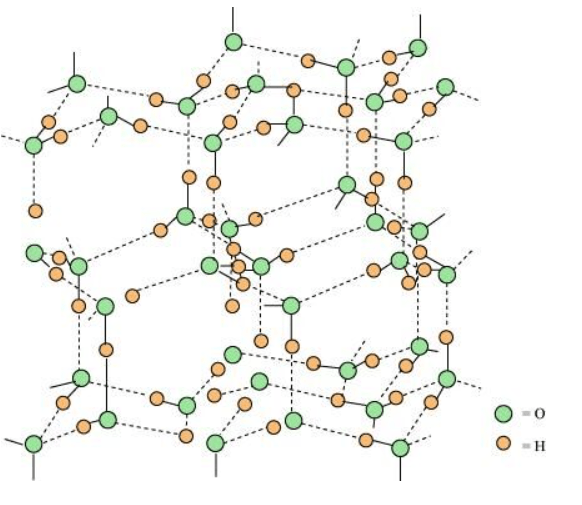The structure is highly ordered and has hydrogen bonding. Each oxygen atom is surrounded tetrahedrally by four other oxygen atoms at a distance of $276 \mathrm{pm}$. The structure also contains wide holes that can hold molecules of appropriate sizes interstitially.

Question 22:What causes the temporary and permanent hardness of water?

Solution. Temporary hardness of water is due to the presence of soluble salts of magnesium and calcium in the form of hydrogen carbonates $\left(\mathrm{MHCO}_{3},\right.$, where $\mathrm{M}=\mathrm{Mg}, \mathrm{Ca})$ in water

Permanent hardness of water is because of the presence of soluble salts of calcium and magnesium in the form of chlorides in water.

Question 23:Discuss the principle and method of softening of hard water by synthetic ion-exchange resins.

Solution. The process of treating permanent hardness of water using synthetic resins is based on the exchange of cations (e.g., $\mathrm{Na}^{+}, \mathrm{Ca}^{2+}, \mathrm{Mg}^{2+}$ etc) and anions $\left(e . g ., \mathrm{Cl}^{-}, \mathrm{SO}_{4}^{2-}, \mathrm{HCO}_{3}^{-}\right.$ etc $)$ present in water by $\mathrm{H}^{+}$ and $\mathrm{OH}^{-}$ ions respectively.

Synthetic resins are of two types:

1) Cation exchange resins

2) Anion exchange resins

Cation exchange resins are large organic molecules that contain the $-\mathrm{SO}_{3} \mathrm{H}$ group. The resin is firstly changed to $\mathrm{RNa}\left(\right.$ from $\left.\mathrm{RSO}_{3} \mathrm{H}\right)$ by treating it with $\mathrm{NaCl}$. This resin then exchanges $\mathrm{Na}^{+}$ ions with $\mathrm{Ca}^{2+}$ and $\mathrm{Mg}^{2+}$ ions, thereby making the water soft.

$2 \mathrm{RNa}+\mathrm{M}^{2+}{ }_{(\alpha q)} \longrightarrow \mathrm{R}_{2} \mathrm{M}_{(s)}+2 \mathrm{Na}^{+}{ }_{\left(\alpha_{8}\right)}$

There are cation exchange resins in $\mathrm{H}^{+}$ form. The resins exchange $\mathrm{H}^{+}$ ions for $\mathrm{Na}^{+}, \mathrm{Ca}^{2+}$, and $\mathrm{Mg}^{2+}$ ions.

$2 \mathrm{RH}+\mathrm{M}^{2+}_{\left(\alpha_{7}\right)} \rightleftarrows \mathrm{MR}_{2(s)}+2 \mathrm{H}_{(\infty)}^{+}$

Anion exchange resins exchange $\mathrm{OH}^{-}$ ions for anions like $\mathrm{Cl}^{-}, \mathrm{HCO}_{3}^{-}$, and $\mathrm{SO}_{4}{ }^{2-}$ present in water.

$\mathrm{RNH}_{2(s)}+\mathrm{H}_{2} \mathrm{O}_{(n)} \rightleftarrows \mathrm{RNH}_{3}^{+} \cdot \mathrm{OH}_{(s)}^{-}$

$\downarrow+\mathrm{X}_{\left(\alpha_{q)}\right.}^{-}$

$\mathrm{RNH}_{3}^{+} \cdot \mathrm{X}_{(s)}^{-}+\mathrm{OH}_{(a q)}^{-}$

During the complete process, water first passes through the cation exchange process. The water obtained after this process is free from mineral cations and is acidic in nature.

This acidic water is then passed through the anion exchange process where $\mathrm{OH}^{-}$ ions neutralize the $\mathrm{H}^{+}$ ions and de-ionize the water obtained.

Question 24:Write chemical reactions to show the amphoteric nature of water.

Solution. The amphoteric nature of water can be described on the basis of the following reactions:

1) Reaction with $\mathrm{H}_{2} \mathrm{~S}$

The reaction takes place as: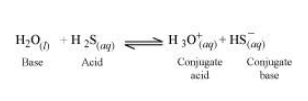In the forward reaction, $\mathrm{H}_{2} \mathrm{O}_{(i)}$ accepts a proton from $\mathrm{H}_{2} \mathrm{~S}_{(a q)} .$ Hence, it acts as a Lewis base.

2) Reaction with $\mathrm{NH}_{3}$

The reaction takes place as: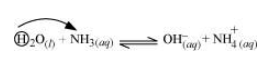In the forward reaction, $\mathrm{H}_{2} \mathrm{O}_{(i)}$ denotes its proton to $\mathrm{NH}_{3(a q)}$. Hence, it acts as a Lewis acid.’

3) Self-ionization of water

In the reaction, two water molecules react as: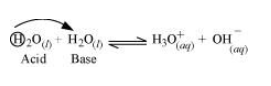Question 25:Write chemical reactions to justify that hydrogen peroxide can function as an oxidizing as well as reducing agent.,

Solution. Hydrogen peroxide, $\mathrm{H}_{2} \mathrm{O}_{2}$ acts as an oxidizing as well as a reducing agent in both acidic and alkaline media.

Reactions involving oxidizing actions are:

1) $2 \mathrm{Fe}^{2+}+2 \mathrm{H}^{+}+\mathrm{H}_{2} \mathrm{O}_{2} \longrightarrow 2 \mathrm{Fe}^{3+}+2 \mathrm{H}_{2} \mathrm{O}$

2) $\mathrm{Mn}^{2+}+\mathrm{H}_{2} \mathrm{O}_{2} \longrightarrow \mathrm{Mn}^{4+}+2 \mathrm{OH}^{-}$

3) $\mathrm{PbS}+4 \mathrm{H}_{2} \mathrm{O}_{2} \longrightarrow \mathrm{PbSO}_{4}+4 \mathrm{H}_{2} \mathrm{O}$

4) $2 \mathrm{Fe}^{2+}+\mathrm{H}_{2} \mathrm{O}_{2} \longrightarrow 2 \mathrm{Fe}^{3+}+2 \mathrm{OH}^{-}$

Reactions involving reduction actions are:

1) $2 \mathrm{MnO}_{4}^{-}+6 \mathrm{H}^{+}+5 \mathrm{H}_{2} \mathrm{O}_{2} \longrightarrow 2 \mathrm{Mn}^{2+}+8 \mathrm{H}_{2} \mathrm{O}+5 \mathrm{O}_{2}$

2) $\mathrm{I}_{2}+\mathrm{H}_{2} \mathrm{O}_{2}+2 \mathrm{OH}^{-} \longrightarrow 2 \mathrm{I}^{-}+2 \mathrm{H}_{2} \mathrm{O}+\mathrm{O}_{2}$

3) $\mathrm{HOCl}+\mathrm{H}_{2} \mathrm{O}_{2} \longrightarrow \mathrm{H}_{3} \mathrm{O}^{+}+\mathrm{Cl}^{-}+\mathrm{O}_{2}$

4) $2 \mathrm{MnO}_{4}^{-}+3 \mathrm{H}_{2} \mathrm{O}_{2} \longrightarrow 2 \mathrm{MnO}_{2}+3 \mathrm{O}_{2}+2 \mathrm{H}_{2} \mathrm{O}+2 \mathrm{OH}^{-}$

Question 26:What is meant by 'demineralised' water and how can it be obtained?

Solution. Demineralised water is free from all soluble mineral salts. It does not contain any anions or cations.

Demineralised water is obtained by passing water successively through a cation exchange (in the $\mathrm{H}^{+}$ form and an anion exchange (in the $\mathrm{OH}^{-}$ form resin

During the cation exchange process, $\mathrm{H}^{+}$ exchanges for $\mathrm{Na}^{+}, \mathrm{Mg}^{2+}, \mathrm{Ca}^{2+}$, and other cations present in water.

$2 \mathrm{RH}_{(s)}+\mathrm{M}_{\left(a_{q}\right)}^{2+} \rightleftarrows \mathrm{MR}_{2(s)}+2 \mathrm{H}_{(a q)}^{+} \ldots \ldots .$ (1)

In the anion exchange process, $\mathrm{OH}^{-}$ exchanges for anions such $\mathrm{as} \mathrm{CO}_{3}^{2-}, \mathrm{SO}_{4}^{2-}, \mathrm{Cl}^{-}, \mathrm{HCO}_{3}^{-}$ etc. present in water.

$\mathrm{RNH}_{2(s)}+\mathrm{H}_{2} \mathrm{O}_{(i)} \rightleftarrows \mathrm{RNH}_{3}^{+} \cdot \mathrm{OH}_{(s)}^{-}$

$\mathrm{RNH}_{3}^{+} \mathrm{OH}_{(s)}^{-}+\mathrm{X}_{(a q)}^{-} \rightleftarrows \mathrm{RNH}_{3}^{+} \cdot \mathrm{X}_{(s)}^{-}+\mathrm{OH}^{-}_{(\text {(q) }} \cdots \ldots \ldots . . .(2)$

$\mathrm{OH}^{-}$ ions liberated in reaction (2) neutralize $\mathrm{H}^{+}$ ions liberated in reaction (1), thereby forming water.

$\mathrm{H}_{(\text {aq) }}^{+}+\mathrm{OH}_{\text {(aq) }}^{-} \longrightarrow \mathrm{H}_{2} \mathrm{O}_{(i)}$

Question 27:Is demineralised or distilled water useful for drinking purposes? If not, how can it be made useful?

Solution. Water is an important part of life. It contains several dissolved nutrients that are required by human beings, plants, and animals for survival. Demineralised water is free of all soluble minerals. Hence, it is not fit for drinking.

It can be made useful only after the addition of desired minerals in specific amounts, which are important for growth

Question 28: Describe the usefulness of water in biosphere and biological systems.

Solution. Water is essential for all forms of life. It constitutes around $65 \%$ of the human body and $95 \%$ of plants. Water plays an important role in the biosphere owing to its high specific heat, thermal conductivity, surface tension, dipole moment, and dielectric constant.

The high heat of vapourization and heat of capacity of water helps in moderating the climate and body temperature of all living beings

It acts as a carrier of various nutrients required by plants and animals for various metabolic reactions.

Question 29:What properties of water make it useful as a solvent? What types of compound can it (i) dissolve, and (ii) hydrolyse?

Solution. A high value of dielectric constants $\left(78.39 \mathrm{C}^{2} / \mathrm{Nm}^{2}\right)$ and dipole moment make water a universal solvent.

Water is able to dissolve most ionic and covalent compounds. Ionic compounds dissolve in water because of the ion-dipole interaction, whereas covalent compounds form hydrogen bonding and dissolve in water.

Water can hydrolyze metallic and non-metallic oxides, hydrides, carbides, phosphides, nitrides and various other salts. During hydrolysis, $\mathrm{H}^{+}$ and $\mathrm{OH}^{-}$ ions of water interact with the reacting molecule.

Some reactions are:

$\mathrm{CaO}+\mathrm{H}_{2} \mathrm{O} \longrightarrow \mathrm{Ca}(\mathrm{OH})_{2}$

$\mathrm{NaH}+\mathrm{H}_{2} \mathrm{O} \longrightarrow \mathrm{NaOH}+\mathrm{H}_{2}$

$\mathrm{CaC}_{2}+\mathrm{H}_{2} \mathrm{O} \longrightarrow \mathrm{C}_{2} \mathrm{H}_{2}+\mathrm{Ca}(\mathrm{OH})_{2}$

Question 30:Knowing the properties of $\mathrm{H}_{2} \mathrm{O}$ and $\mathrm{D}_{2} \mathrm{O}$, do you think that $\mathrm{D}_{2} \mathrm{O}$ can be used for drinking purposes?

Solution. Heavy water $\left(\mathrm{D}_{2} \mathrm{O}\right)$ acts as a moderator, i.e., it slows the rate of a reaction. Due to this property of $\mathrm{D}_{2} \mathrm{O}$, it cannot be used for drinking purposes because it will slow down anabolic and catabolic reactions taking place in the body and lead to a casualty.

Question 31:What is the difference between the terms 'hydrolysis' and 'hydration'?

Solution. Hydrolysis is defined as a chemical reaction in which hydrogen and hydroxide ions $\left(\mathrm{H}^{+}\right.$ and $\mathrm{OH}^{-}$ ions) of water molecule react with a compound to form products. For example:

$\mathrm{NaH}+\mathrm{H}_{2} \mathrm{O} \longrightarrow \mathrm{NaOH}+\mathrm{H}_{2}$

Hydration is defined as the addition of one or more water molecules to ions or molecules to form hydrated compounds. For example:

$\mathrm{CuSO}_{4}+5 \mathrm{H}_{2} \mathrm{O} \longrightarrow \mathrm{CuSO}_{4} \cdot 5 \mathrm{H}_{2} \mathrm{O}$

Question 32:How can saline hydrides remove traces of water from organic compounds?

Solution. Saline hydrides are ionic in nature. They react with water to form a metal hydroxide along with the liberation of hydrogen gas. The reaction of saline hydrides with water can be represented as:

$\mathrm{AH}_{(s)}+\mathrm{H}_{2} \mathrm{O}_{(f)} \longrightarrow \mathrm{AOH}_{(a q)}+\mathrm{H}_{2(g)}$

$($ where, $A=N a, C a, \ldots \ldots)$

When added to an organic solvent, they react with water present in it. Hydrogen escapes into the atmosphere leaving behind the metallic hydroxide. The dry organic solvent distills over.

Question 33:What do you expect the nature of hydrides is, if formed by elements of atomic

numbers $15,19,23$ and 44 with dihydrogen? Compare their behaviour towards water.

Solution. The elements of atomic numbers $15,19,23$, and 44 are phosphorus, potassium, vanadium, and ruthenium respectively.

1) Hydride of phosphorus

Hydride of nitrogen $\left(\mathrm{PH}_{3}\right)$ is a covalent molecule. It is an electron-rich hydride owing to the presence of excess electrons as a lone pair on phosphorus.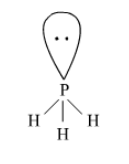2) Hydride of potassium

Dihydrogen forms an ionic hydride with potassium owing to the high electropositive nature of potassium. It is crystalline and non-volatile in nature

3) Hydrides of Vanadium and Ruthenium

Both vanadium and ruthenium belong to the $d$ -block of the periodic table. The metals of $d$ -block form metallic or non-stoichiometric hydrides. Hydrides of vanadium and ruthenium are therefore, metallic in nature having a deficiency of hydrogen.

4) Behaviour of hydrides towards water

Potassium hydride reacts violently with water as:

$\mathrm{KH}_{(s)}+\mathrm{H}_{2} \mathrm{O}_{(a)} \longrightarrow \mathrm{KOH}_{\left(a_{q}\right)}+\mathrm{H}_{2(g)}$

Phosphorus $\left(\mathrm{PH}_{3}\right)$ is covalent hydride and slightly soluble in water.

Hydrides of vanadium and Ruthenium do not react with water. Hence, the increasing order of reactivity of the hydrides is $(\mathrm{V}, \mathrm{Ru}) \mathrm{H}<\mathrm{NH}_{3}<\mathrm{KH}$.

Question $34$Do you expect different products in solution when aluminium (III) chloride and potassium chloride treated separately with (i) normal water (ii) acidified water, and(iii) alkaline water? Write equations wherever necessary.

Solution. Potassium chloride $(\mathrm{KCl})$ is the salt of a strong acid $(\mathrm{HCl})$ and strong base $(\mathrm{KOH}) .$ Hence, it is neutral in nature and does not undergo hydrolysis in normal water. It dissociates into ions as follows.

$\mathrm{KCl}_{(s)} \stackrel{\text { water }}{\longrightarrow} \mathrm{K}_{(a q)}^{+}+\mathrm{Cl}_{(a q)}^{-}$

In acidified and alkaline water, the ions do not react and remain as such.

Aluminium (III) chloride is the salt of a strong acid $(\mathrm{HCl})$ and weak base $\left[\mathrm{Al}(\mathrm{OH})_{3}\right]$. Hence, it undergoes hydrolysis in normal water.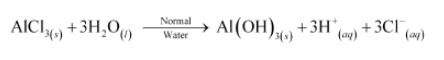In acidified water, $\mathrm{H}^{+}$ ions react with $\mathrm{Al}(\mathrm{OH})_{3}$ forming water and giving $\mathrm{Al}^{3+}$ ions. Hence, in acidified water, $\mathrm{AICl}_{3}$ will exist as $\mathrm{Al}^{3+}{ }_{(a q)}$ and $\mathrm{Cl}_{\left(a_{q}\right)}^{-}$ ions

$\mathrm{AlCl}_{3(s)} \underset{\text { Wilifed }}{\longrightarrow} \mathrm{Al}^{3+}{ }_{\left(a_{q}\right)}+3 \mathrm{Cl}^{-}{ }_{(a q)}$

In alkaline water, the following reaction takes place:

$\mathrm{Al}(\mathrm{OH})_{3(s)}+\underbrace{\mathrm{OH}_{(a q)}^{-}}_{\text {from alkaline water }} \longrightarrow\left[\mathrm{Al}(\mathrm{OH})_{4}\right]_{(a)}^{-}+2 \mathrm{H}_{2} \mathrm{O}_{(t)}$

Question 35:How does $\mathrm{H}_{2} \mathrm{O}_{2}$ behave as a bleaching agent?

Solution. $\mathrm{H}_{2} \mathrm{O}_{2}$ or hydrogen peroxide acts as a strong oxidizing agent both in acidic and basic media.

When added to a cloth, it breaks the chemical bonds of the chromophores (colour producing agents). Hence, the visible light is not absorbed and the cloth gets whitened.

Question 36:What do you understand by the terms:

(i) hydrogen economy (ii) hydrogenation (iii) 'syngas' (iv) water-gas shift reaction (v) fuel-cell?

Solution. (i) Hydrogen economy

Hydrogen economy is a technique of using dihydrogen in an efficient way. It involves transportation and storage of dihydrogen in the form of liquid or gas.

Dihydrogen releases more energy than petrol and is more eco-friendly. Hence, it can be used in fuel cells to generate electric power. Hydrogen economy is about the transmission of this energy in the form of dihydrogen.

(ii) Hydrogenation

Hydrogenation is the addition of dihydrogen to another reactant. This process is used to reduce a compound in the presence of a suitable catalyst. For example, hydrogenation of vegetable oil using nickel as a catalyst gives edible fats such as vanaspati, ghee etc.

(iii) Syngas

Syngas is a mixture of carbon monoxide and dihydrogen. Since the mixture of the two gases is used for the synthesis of methanol, it is called syngas, synthesis gas, or water gas.

Syngas is produced on the action of steam with hydrocarbons or coke at a high temperature in the presence of a catalyst.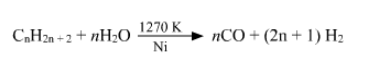For example: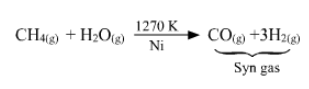(iv) Water shift reaction

It is a reaction of carbon monoxide of syngas mixture with steam in the presence of a catalyst as: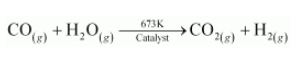This reaction is used to increase the yield of dihydrogen obtained from the coal gasification reaction as: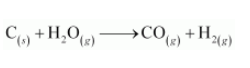(v) Fuel cells

fuels because it is eco-friendly and releases greater energy per unit mass of fuel as compared to gasoline and other fuels.